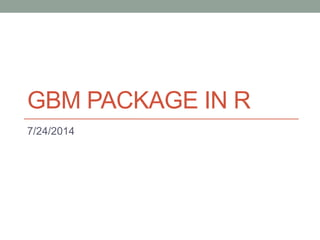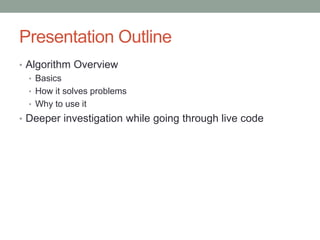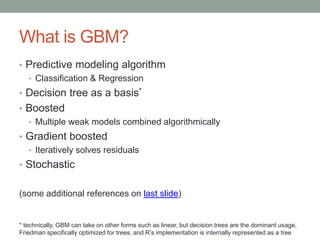Ce diaporama a bien été signalé.
Le téléchargement de votre SlideShare est en cours. ×

# GBM package in rChargement dans…3
×

1 sur 21
1 sur 21

# GBM package in r

Presentation delivered to Austin R user group on 7/24/2014: GBM package in R, by Mark Landry.

Presentation delivered to Austin R user group on 7/24/2014: GBM package in R, by Mark Landry.

## Plus De Contenu Connexe

### GBM package in r

1. 1. GBM PACKAGE IN R 7/24/2014
2. 2. Presentation Outline • Algorithm Overview • Basics • How it solves problems • Why to use it • Deeper investigation while going through live code
3. 3. What is GBM? • Predictive modeling algorithm • Classification & Regression • Decision tree as a basis* • Boosted • Multiple weak models combined algorithmically • Gradient boosted • Iteratively solves residuals • Stochastic (some additional references on last slide) * technically, GBM can take on other forms such as linear, but decision trees are the dominant usage, Friedman specifically optimized for trees, and R’s implementation is internally represented as a tree
4. 4. Predictive Modeling Landscape: General Purpose Algorithms (forillustrativepurposes only,nottoscale,precise,orcomprehensive;author’sperspective) Linear Models Decision Trees Others Linear Models (lm) Generalized Linear Models (glm) Regularized Linear Models (glmnet) Classification And Regression Trees (rpart) Random Forest (randomForest) Gradient Boosted Machines (gbm) Nearest Neighbor (kNN) Neural Networks (nnet) Support Vector Machines (kernlab) complexity Naïve Bayes (klaR) Splines (earth) More Comprehensive List: http://caret.r-forge.r-project.org/modelList.html
5. 5. GBM’s decision tree structure
6. 6. Why GBM? • Characteristics • Competitive Performance • Robust • Loss functions • Fast (relatively) • Usages • Quick modeling • Variable selection • Final-stage precision modeling
7. 7. Competitive Performance • Competitive with high-end algorithms such as RandomForest • Reliable performance • Avoids nonsensical predictions • Rare to produce worse predictions than simpler models • Often in winning Kaggle solutions • Cited within winning solution descriptions in numerous competitions, including \$3M competition • Many of the highest ranked competitors use it frequently • Used in 4 of 5 personal top 20 finishes
8. 8. Robust • Explicitly handles NAs • Scaling/normalization is unnecessary • Handles more factor levels than random forest (1024 vs 32) • Handles perfectly correlated independent variables • No [known] limit to number of independent variables
9. 9. Loss Functions • Gaussian: squared loss • Laplace: absolute loss • Bernoulli: logistic, for 0/1 • Huberized: hinge, for 0/1 • Adaboost: exponential loss, for 0/1 • Multinomial: more than one class (produces probability matrix) • Quantile: flexible alpha (e.g. optimize for 2 StDev threshold) • Poisson: Poisson distribution, for counts • CoxPH: Cox proportional hazard, for right-censored • Tdist: t-distribution loss • Pairwise: rankings (e.g. search result scoring) • Concordant pairs • Mean reciprocal rank • Mean average precision • Normalized discounted cumulative gain
10. 10. Drawbacks • Several hyper-parameters to tune • I typically use roughly the same parameters to start, unless I suspect the data set might have peculiar characteristics • For creating a final model, tuning several parameters is advisable • Still has capacity to overfit • Despite internal cross-validation, it is still particularly prone to overfit ID-like columns (suggestion: withhold them) • Can have trouble with highly noisy data • Black box • However, GBM package does provide tools to analyze the resulting models
11. 11. Deeper Analysis via Walkthrough • Hyper-parameter explanations (some, not all) • Quickly analyze performance • Analyze influence of variables • Peek under the hood…then follow a toy problem For those not attending the presentation, the code at the back is run at this point and discussed. The remaining four slides were mainly to supplement the discussion of the code and comments, and there was not sufficient time.
12. 12. Same analysis with a simpler data set Note that one can recreate the predictions of this first tree by finding the terminal node for any prediction and using the Prediction value (final column in data frame). Those values for all desired trees, plus the initial value (mean for this) is the prediction. Matches predictions 1 & 3 Matches predictions 2,4 & 5
13. 13. Same analysis with a simpler data set Explanation 1 tree built. Tree has one decision only, node 0. Node 0 indicates it split the 3rd field (SplitVar:2), to where values below 1.5 (ordered values 0 & 1 which are a & b) went to node 1; values above 1.5 (2/3 = c/d) went to node 2; missing (none) go to node 3. Node 1 (X3=A/B) is a terminal node (SplitVar -1) and it predicts the mean plus -0.925. Node 2 (X3=C/D) is a terminal node and it predicts the mean plus 1.01. Node 3 (none) is a terminal node and it predicts the mean plus 0, effectively. Later saw that gbm1\$initF will show the intercept, which in this case is the mean.
14. 14. GBM predict: fit a GBM to data • gbm(formula = formula(data), • distribution = "bernoulli", • n.trees = 100, • interaction.depth = 1, • n.minobsinnode = 10, • shrinkage = 0.001, • bag.fraction = 0.5, • train.fraction = 1.0, • cv.folds=0, • weights, • data = list(), • var.monotone = NULL, • keep.data = TRUE, • verbose = "CV", • class.stratify.cv=NULL, • n.cores = NULL)
15. 15. Effect of shrinkage & trees Source: https://www.youtube.com/watch?v=IXZKgIsZRm0 (GBM explanation by SciKit author)
16. 16. Code Dump • The code has been copied from a text R script into PowerPoint, so the format isn’t great, but it should look OK if copying and pasting back out to a text file. If not, here it is on Github. • The code shown uses a competition data set that is comparable to real world data and uses a simple GBM to predict sale prices of construction equipment at auction. • A GBM model was fit against 100k rows with 45-50 variables in about 2-4 minutes during the presentation. It improves the RMSE of prediction against the mean from ~24.5k to ~9.7k, when scored on data the model had not seen (and future dates, so the 100k/50k splits should be valid), with fairly stable train:test performance. • After predictions are made and scored, some GBM utilities are used to see which variables the model found most influential, see how the top 2 variables are used (per factor for one; throughout a continuous distribution for the other), and see interaction effects of specific variable pairs. • Note: GBM was used by my teammate and I to finish 12th out of 476 in this competition (albeit a complex ensemble of GBMs)
17. 17. Code Dump: Page1 library(Metrics) ##load evaluation package setwd("C:/Users/Mark_Landry/Documents/K/dozer/") ##Done in advance to speed up loading of data set train<-read.csv("Train.csv") ## Kaggle data set: http://www.kaggle.com/c/bluebook-for-bulldozers/data train\$saleTransform<-strptime(train\$saledate,"%m/%d/%Y %H:%M") train<-train[order(train\$saleTransform),] save(train,file="rTrain.Rdata") load("rTrain.Rdata") xTrain<-train[(nrow(train)-149999):(nrow(train)-50000),5:ncol(train)] xTest<-train[(nrow(train)-49999):nrow(train),5:ncol(train)] yTrain<-train[(nrow(train)-149999):(nrow(train)-50000),2] yTest<-train[(nrow(train)-49999):nrow(train),2] dim(xTrain); dim(xTest) sapply(xTrain,function(x) length(levels(x))) ## check levels; gbm is robust, but still has a limit of 1024 per factor; for initial model, remove ## after iterating through model, would want to go back and compress these factors to investigate ## their usefulness (or other information analysis) xTrain\$saledate<-NULL; xTest\$saledate<-NULL xTrain\$fiModelDesc<-NULL; xTest\$fiModelDesc<-NULL xTrain\$fiBaseModel<-NULL; xTest\$fiBaseModel<-NULL xTrain\$saleTransform<-NULL; xTest\$saleTransform<-NULL
18. 18. Code Dump: Page2 library(gbm) ## Set up parameters to pass in; there are many more hyper-parameters available, but these are the most common to control GBM_NTREES = 400 ## 400 trees in the model; can scale back later for predictions, if desired or overfitting is suspected GBM_SHRINKAGE = 0.05 ## shrinkage is a regularization parameter dictating how fast/aggressive the algorithm moves across the loss gradient ## 0.05 is somewhat aggressive; default is 0.001, values below 0.1 tend to produce good results ## decreasing shrinkage generally improves results, but requires more trees, so the two should be adjusted in tandem GBM_DEPTH = 4 ## depth 4 means each tree will evaluate four decisions; ## will always yield [3*depth + 1] nodes and [2*depth + 1] terminal nodes (depth 4 = 9) ## because each decision yields 3 nodes, but each decision will come from a prior node GBM_MINOBS = 30 ## regularization parameter to dictate how many observations must be present to yield a terminal node ## higher number means more conservative fit; 30 is fairly high, but good for exploratory fits; default is 10 ## Fit model g<-gbm.fit(x=xTrain,y=yTrain,distribution = "gaussian",n.trees = GBM_NTREES,shrinkage = GBM_SHRINKAGE, interaction.depth = GBM_DEPTH,n.minobsinnode = GBM_MINOBS) ## gbm fit; provide all remaining independent variables in xTrain; provide targets as yTrain; ## gaussian distribution will optimize squared loss;
19. 19. Code Dump: Page3 ## get predictions; first on train set, then on unseen test data tP1 <- predict.gbm(object = g,newdata = xTrain,GBM_NTREES) hP1 <- predict.gbm(object = g,newdata = xTest,GBM_NTREES) ## compare model performance to default (overall mean) rmse(yTrain,tP1) ## 9452.742 on data used for training rmse(yTest,hP1) ## 9740.559 ~3% drop on unseen data; does not seem to be overfit rmse(yTest,mean(yTrain)) ## 24481.08 overall mean; cut error rate (from perfection) by 60% ## look at variables summary(g) ## summary will plot and then show the relative influence of each variable to the entire GBM model (all trees) ## test dominant variable mean library(sqldf) trainProdClass<-as.data.frame(cbind(as.character(xTrain\$fiProductClassDesc),yTrain)) testProdClass<-as.data.frame(cbind(as.character(xTest\$fiProductClassDesc),yTest)) colnames(trainProdClass)<-c("fiProductClassDesc","y"); colnames(testProdClass)<-c("fiProductClassDesc","y") ProdClassMeans<-sqldf("SELECT fiProductClassDesc,avg(y) avg, COUNT(*) n FROM trainProdClass GROUP BY fiProductClassDesc") ProdClassPredictions<-sqldf("SELECT case when n > 30 then avg ELSE 31348.63 end avg FROM ProdClassMeans P LEFT JOIN testProdClass t ON t.fiProductClassDesc = P.fiProductClassDesc") rmse(yTest,ProdClassPredictions\$avg) ## 29082.64 ? peculiar result on the fiProductClassDesc means, which seemed fairly stable and useful ##seems to say that the primary factor alone is not helpful; full tree needed
20. 20. Code Dump: Page4 ## Investigate actual GBM model pretty.gbm.tree(g,1) ## show underlying model for the first decision tree summary(xTrain[,10]) ## underlying model showed variable 9 to be first point in tree (9 with 0 index = 10th column) g\$initF ## view what is effectively the "y intercept" mean(yTrain) ## equivalence shows gaussian y intercept is the mean t(g\$c.splits[]) ## show whether each factor level should go left or right plot(g,10) ## plot fiProductClassDesc, the variable with the highest rel.inf plot(g,3) ## plot YearMade, continuous variable with 2nd highest rel.inf interact.gbm(g,xTrain,c(10,3)) ## compute H statistic to show interaction; integrates interact.gbm(g,xTrain,c(10,3)) ## example of uninteresting interaction
21. 21. Selected References • CRAN • Documentation • vignette • Algorithm publications: • Greedy function approximation: A gradient boosting machine Friedman 2/99 • Stochastic Gradient Boosting; Friedman 3/99 • Overviews • Gradient boosting machines, a tutorial: Frontiers (4/13) • Wikipedia (pretty good article, really) • Video of author of GBM in Python: Gradient Boosted Regression Trees in scikit-learn • Very helpful, but the implementation is not decision “stumps” in R, so some things are different in R (e.g. number of trees need not be so high)